Flutter之初识贝塞尔曲线 - 实现炫酷的路由动画

前言

Flutter炫酷的波纹路由动画第一眼望过去，的确挺炫酷的，目测就比较有难度，反正Flutter有热重载，首先就开始用用最基本的组件一点一点试。

• （1） 观察上面的动画，在动画到一半也就是中间有一条直线的时候，不难想象，一个圆的可以大到在手机屏幕内呈直线的话，那么这个圆的dp应该得上 100,000（测试中的数据），而且永远都不能达到绝对的直线。

• （2）即使在视觉上给人了直线的感觉，抛开性能的开销，从动画的层面分析，圆的半径就是从一个按钮的大小（假设50dp）扩大到 100,000 ，让人感知的数据范围也仅仅在 50dp~1000dp ，而这部分的数据仅仅占用整个动画的 1% ,所以这个动画的时长是极其难控制的。

• 余弦定理
• 贝塞尔曲线
• 空间坐标点的旋转

一、认识贝塞尔曲线

• 一阶贝塞尔曲线（2 个控制点）
• 二阶贝塞尔曲线（3 个控制点）
• 三阶贝塞尔曲线（4 个控制点）
• n阶贝塞尔曲线（n+1个控制点）

1、一阶贝塞尔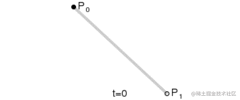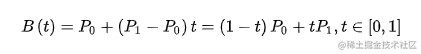2、二阶贝塞尔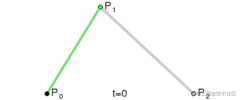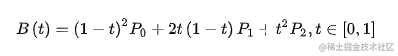3、三阶贝塞尔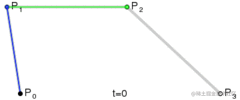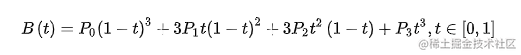二、使用贝塞尔曲线画圆

1、画出近似 1/4 圆弧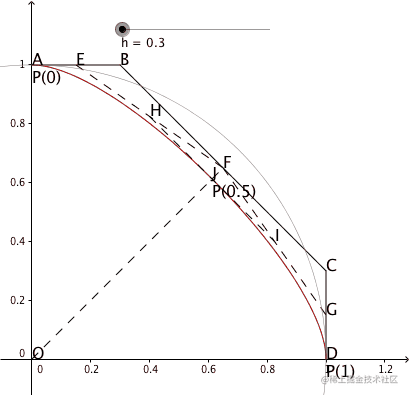double h = (math.sqrt(2) - 1.0) * 4.0 / 3.0;

(0,1)
(h,1)
(1,h)
(1,0))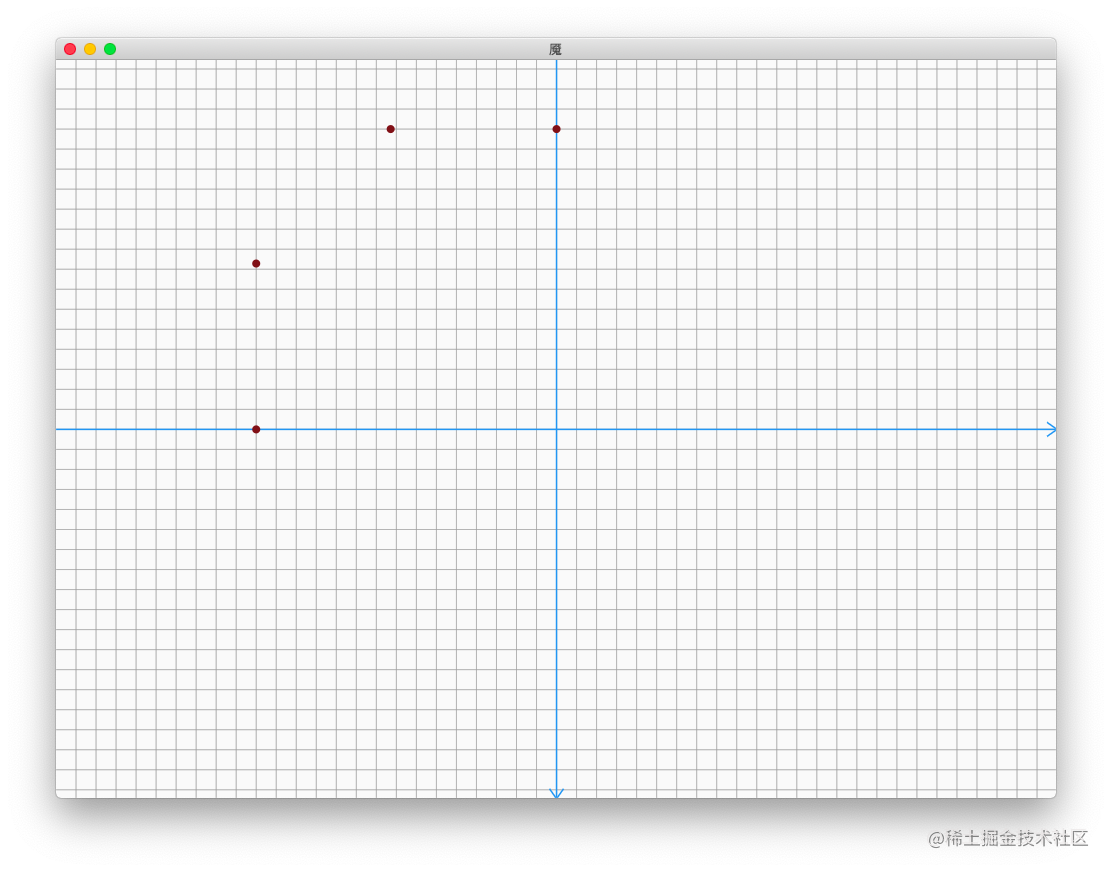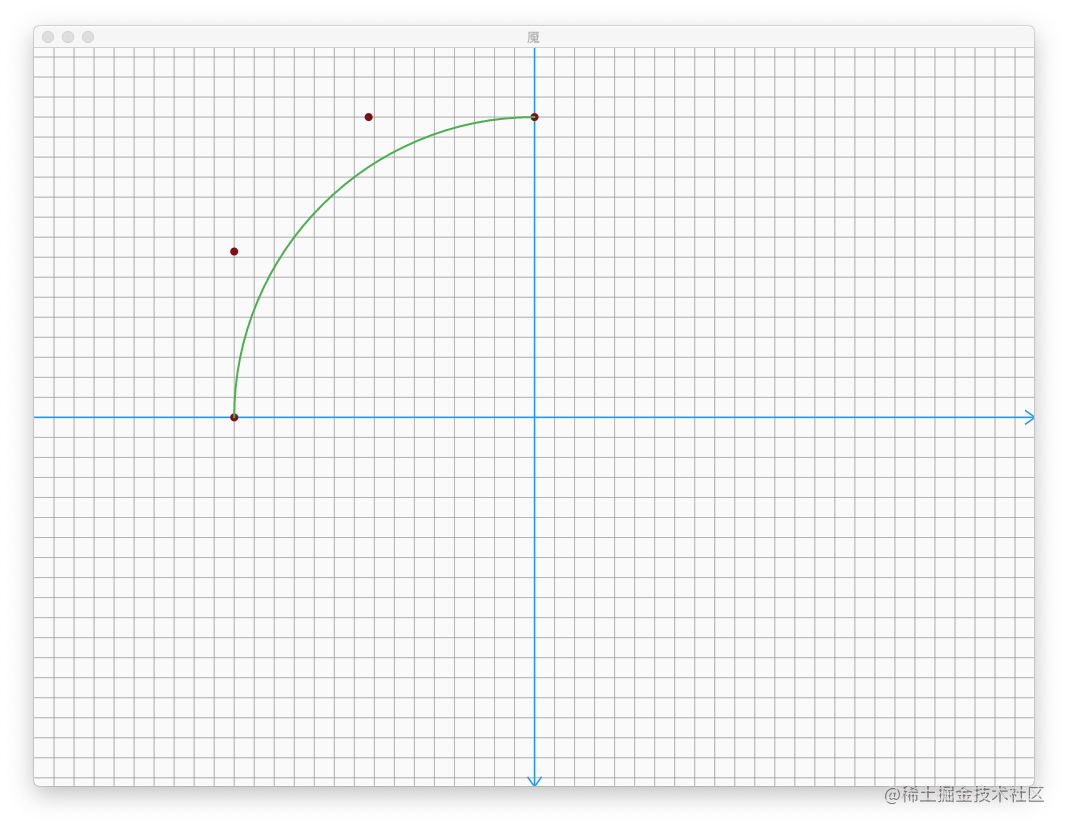2、拼接成整个圆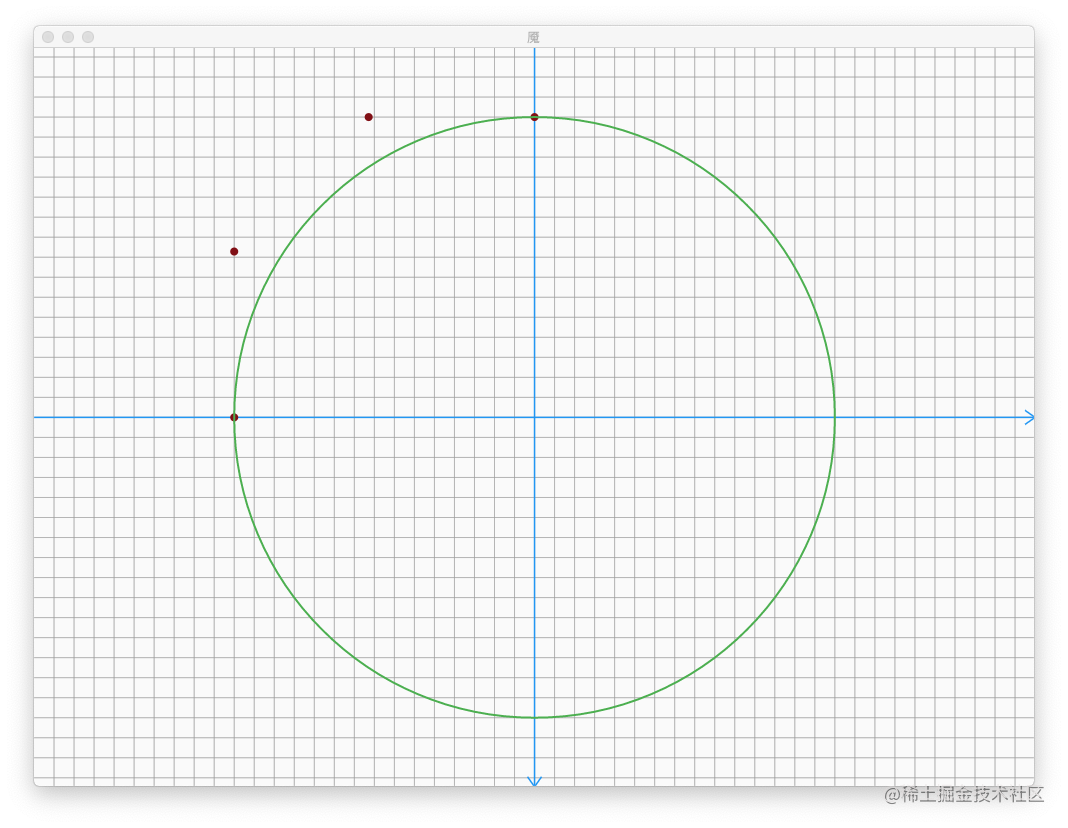final List<Offset> _firstControllerPoints = <Offset>[];
final List<Offset> _secondControllerPoints = <Offset>[];
final List<Offset> _thirdControllerPoints = <Offset>[];
final List<Offset> _fourthControllerPoints = <Offset>[];
double h = (math.sqrt(2) - 1.0) * 4.0 / 3.0;
void generateControllerPoints(Offset circelCenter, double circleRadius) {
// ------------------------------
circelCenter.dy,
));
circelCenter.dy - h,
));
circelCenter.dx - h,
));
circelCenter.dx,
));
// ------------------------------
circelCenter.dx,
));
circelCenter.dx + h,
));
circelCenter.dy - h,
));
circelCenter.dy,
));
// ------------------------------
circelCenter.dy,
));
circelCenter.dy + h,
));
circelCenter.dx + h,
));
circelCenter.dx,
));
// ------------------------------
circelCenter.dx,
));
circelCenter.dx - h,
));
circelCenter.dy + h,
));
circelCenter.dy,
));
}
Path getCirclePath() {
final Path path = Path();
path.moveTo(
_firstControllerPoints.dx,
_firstControllerPoints.dy,
);
path.cubicTo(
_firstControllerPoints.dx,
_firstControllerPoints.dy,
_firstControllerPoints.dx,
_firstControllerPoints.dy,
_firstControllerPoints.dx,
_firstControllerPoints.dy,
);
path.cubicTo(
_secondControllerPoints.dx,
_secondControllerPoints.dy,
_secondControllerPoints.dx,
_secondControllerPoints.dy,
_secondControllerPoints.dx,
_secondControllerPoints.dy,
);
path.cubicTo(
_thirdControllerPoints.dx,
_thirdControllerPoints.dy,
_thirdControllerPoints.dx,
_thirdControllerPoints.dy,
_thirdControllerPoints.dx,
_thirdControllerPoints.dy,
);
path.cubicTo(
_fourthControllerPoints.dx,
_fourthControllerPoints.dy,
_fourthControllerPoints.dx,
_fourthControllerPoints.dy,
_fourthControllerPoints.dx,
_fourthControllerPoints.dy,
);
return path;
}

三、动画的实现

1、动画的分割

• 1.由一个小圆逐渐变大，高度最值可以刚好撑满屏幕。
• 2.将 1/4 的圆弧逐渐拉直，模拟圆继续扩大的效果。

• 1.将直线逐渐向 1/4 的圆弧过渡。
• 2.由一个大圆逐渐变小。

2、实现第一部分动画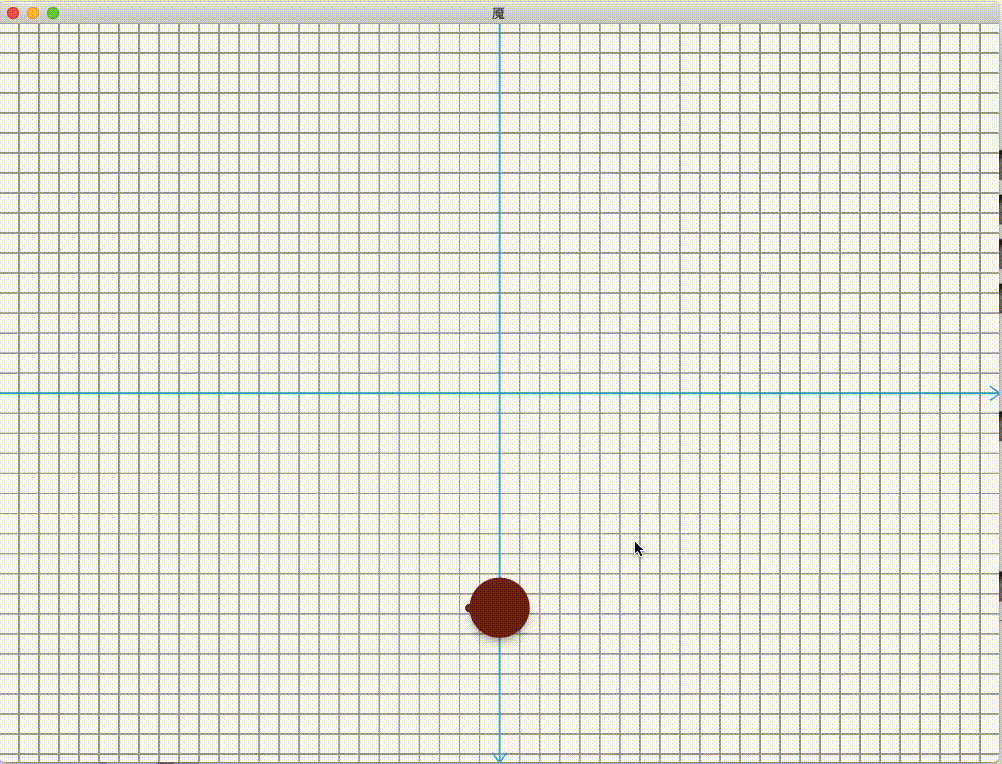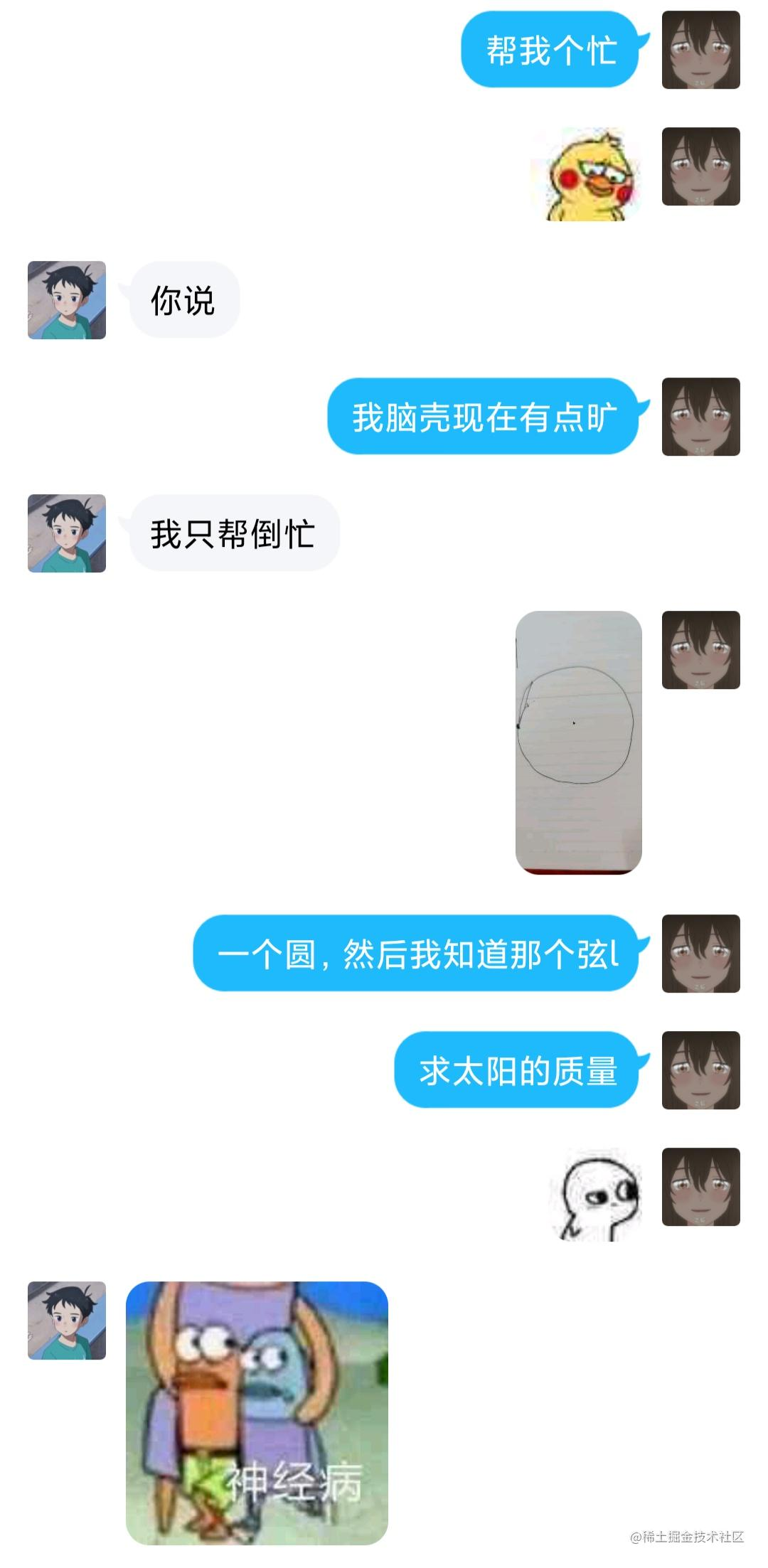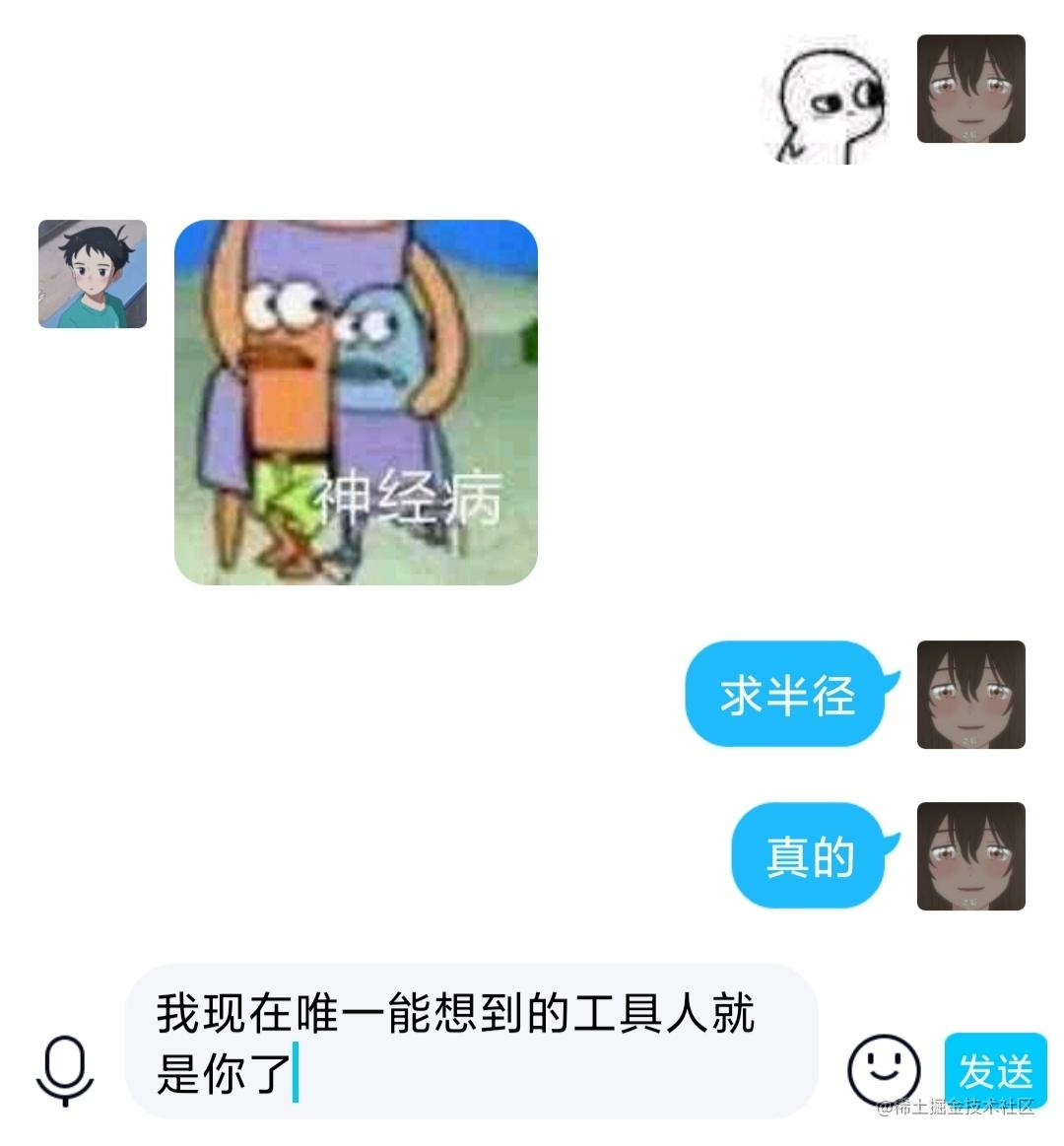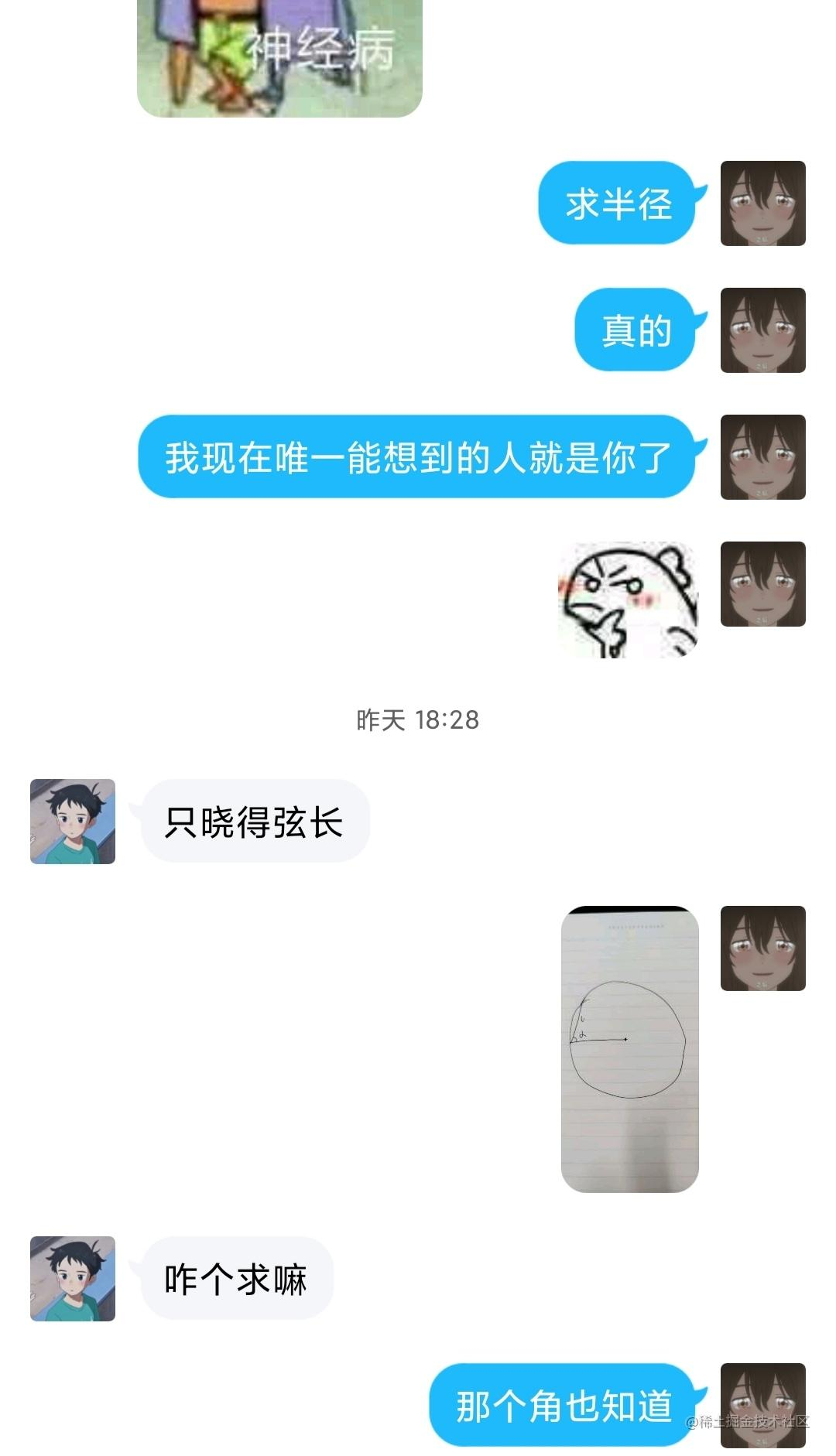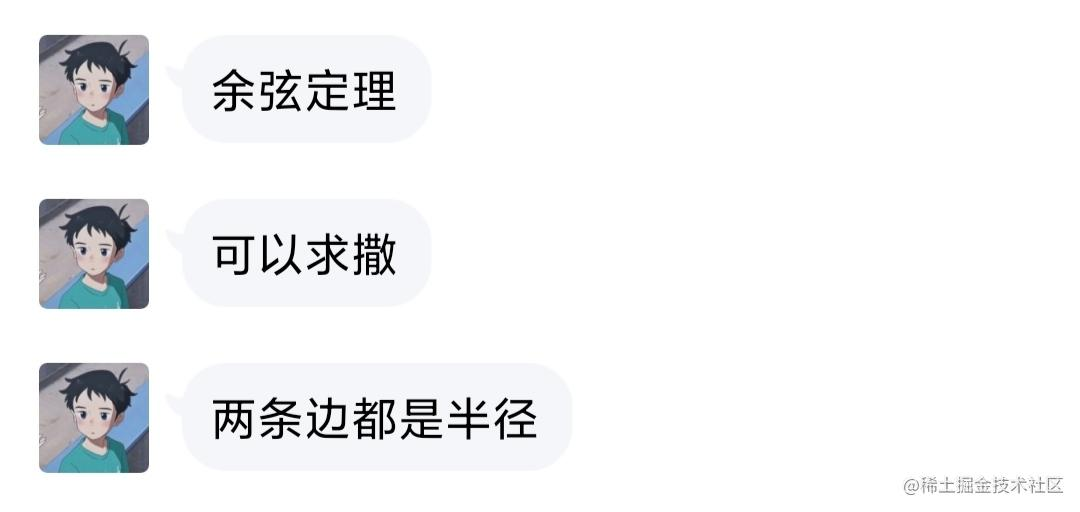• 按钮中心坐标到屏幕端点的距离
• 按钮中心坐标到与屏幕端点的向量的弧度值

Offset point1,
Offset point2,
) {
point1 = Offset(point1.dx, -point1.dy);
point2 = Offset(point2.dx, -point2.dy);
double r;
final Offset line = point2 - point1;
final double k = line.dy / line.dx;
final double angle = math.atan(k);
print('line向量坐标为====>\$line');
final double cosx = math.cos(angle);
print('cosx====>\$cosx');
final double sinx = math.sin(angle);
print('sinx====>\$sinx');
final double cos2x = math.pow(cosx, 2).toDouble() - math.pow(sinx, 2);
final double l = line.distance;
r = math.sqrt(math.pow(l, 2) / (2 * (1 + cos2x)));
return r;
}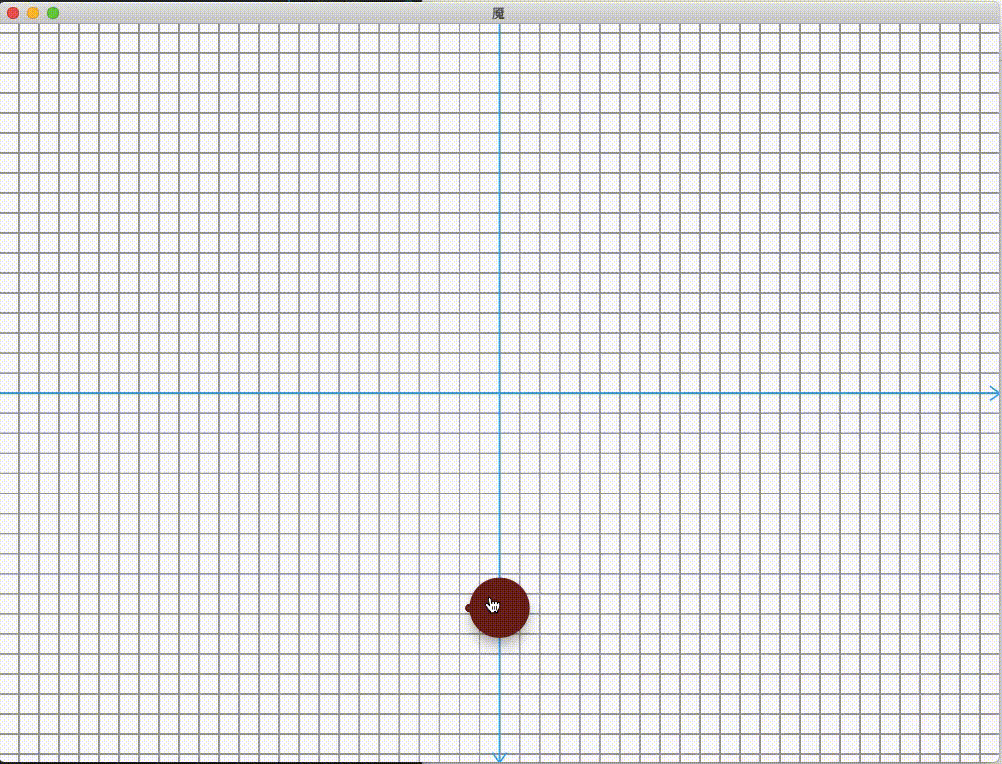3、实现第二部分动画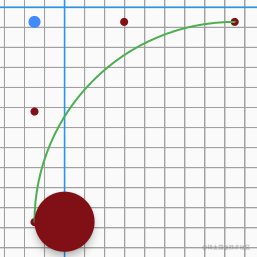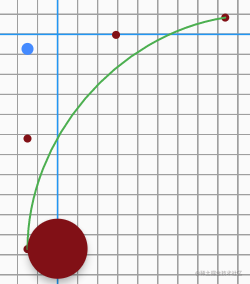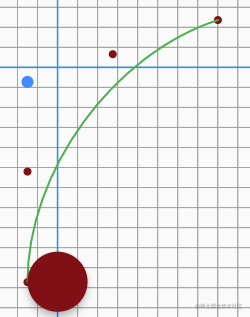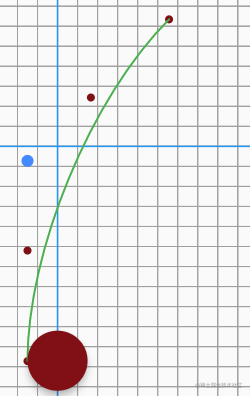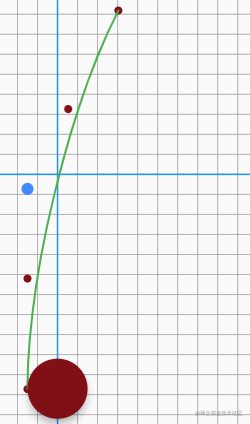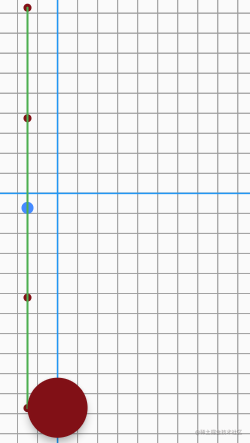double getNegative(num number) {
if (number.isNegative) {
return -1;
}
return 1;
}

double getVectorInitAngle(Offset vector) {
if (vector.dx == 0) {
if (vector.dy > 0) {
return math.pi / 2;
} else {
return math.pi * 3 / 2;
}
}
if (vector.dy == 0) {
if (vector.dx > 0) {
return 0;
} else {
return math.pi;
}
}
if (vector.dx > 0 && vector.dy > 0) {
return math.atan2(vector.dx, vector.dy);
// print(math.atan2(vector.dx, vector.dy) * 180 / math.pi);
} else if (vector.dx < 0 && vector.dy > 0) {
return math.atan2(vector.dx, vector.dy) + math.pi;
// print(180 + math.atan2(vector.dx, vector.dy) * 180 / math.pi);
} else if (vector.dx < 0 && vector.dy < 0) {
return math.pi / 2 - math.atan2(vector.dx, vector.dy);
// print(90 - math.atan2(vector.dx, vector.dy) * 180 / math.pi);
} else if (vector.dx > 0 && vector.dy < 0) {
return math.atan2(vector.dx, vector.dy) + math.pi;
// print(180 + math.atan2(vector.dx, vector.dy) * 180 / math.pi);
}
return 0;
}

Offset rotateOffset(Offset point, double angle, [Offset origin]) {
Offset tmp;
origin ??= const Offset(0, 0);
final Offset vector = point - origin;
if (angle == 0.0) {
return point;
}
print('vector====\$vector===初始角度===>\${getVectorInitAngle(vector)}');
print('angle====\${angle * 180 / math.pi}');
tmp = Offset(
origin.dx +
vector.distance * math.cos(angle + getVectorInitAngle(vector)),
origin.dy +
vector.distance * math.sin(angle + getVectorInitAngle(vector)),
);
return tmp;
}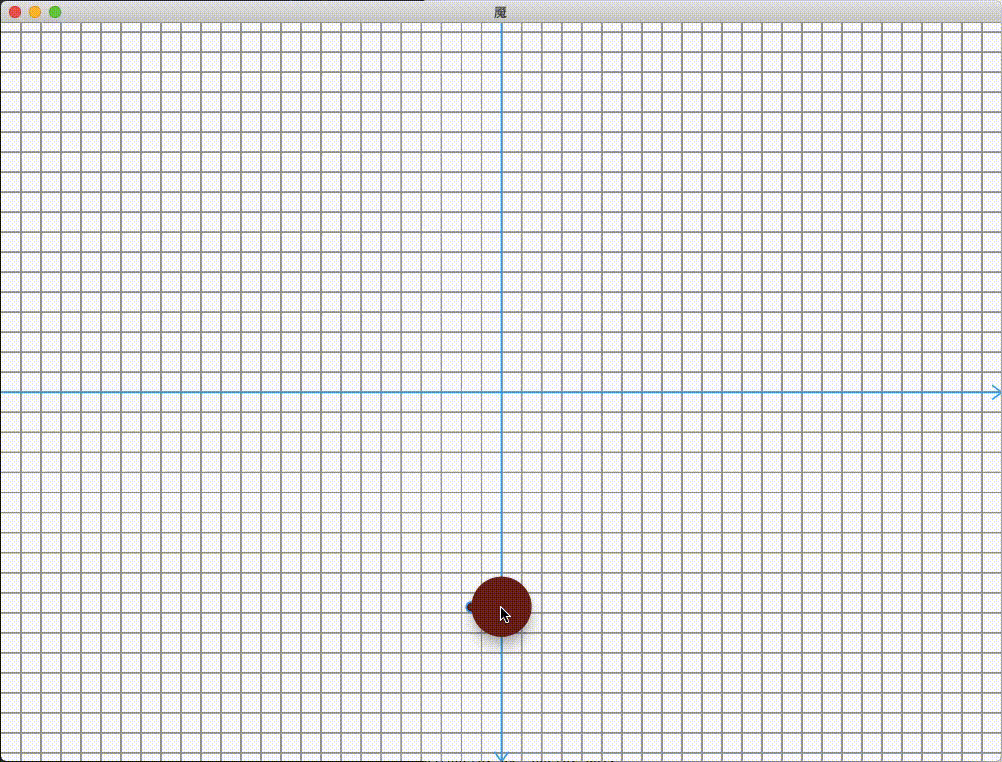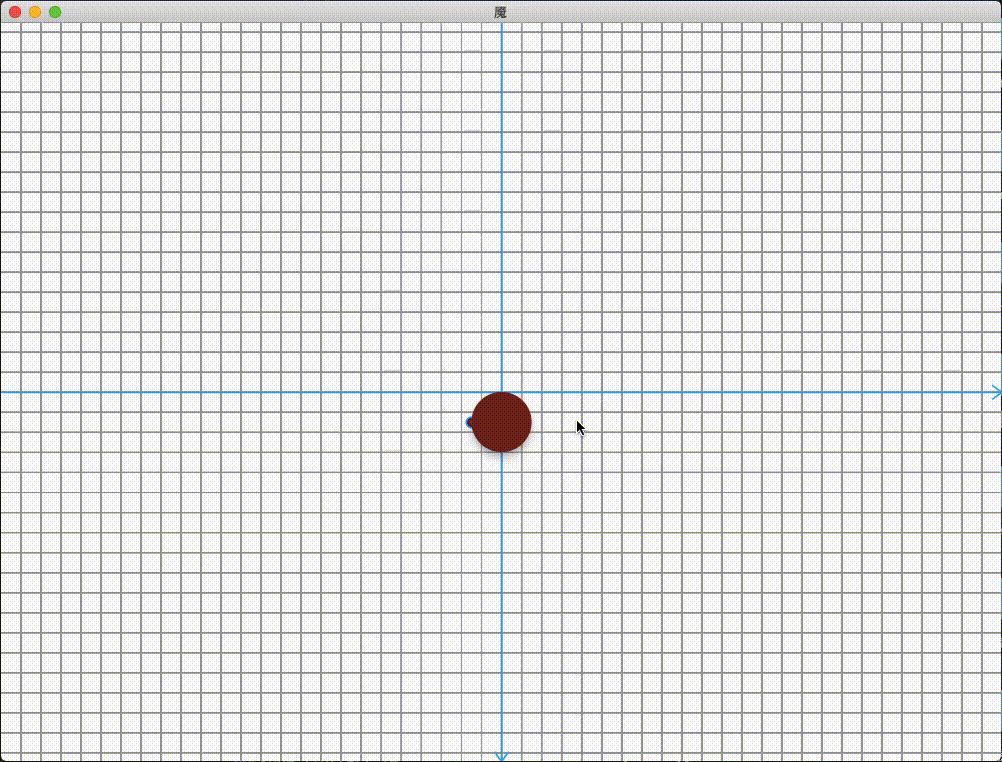• 第二个圆弧的控制点的初位置是用的第一个圆弧的终点，以此类推。
• 在第二部分的动画中，部分点随一个固定点旋转后，导致使用了这个点的贝塞尔发生预期之外的变化。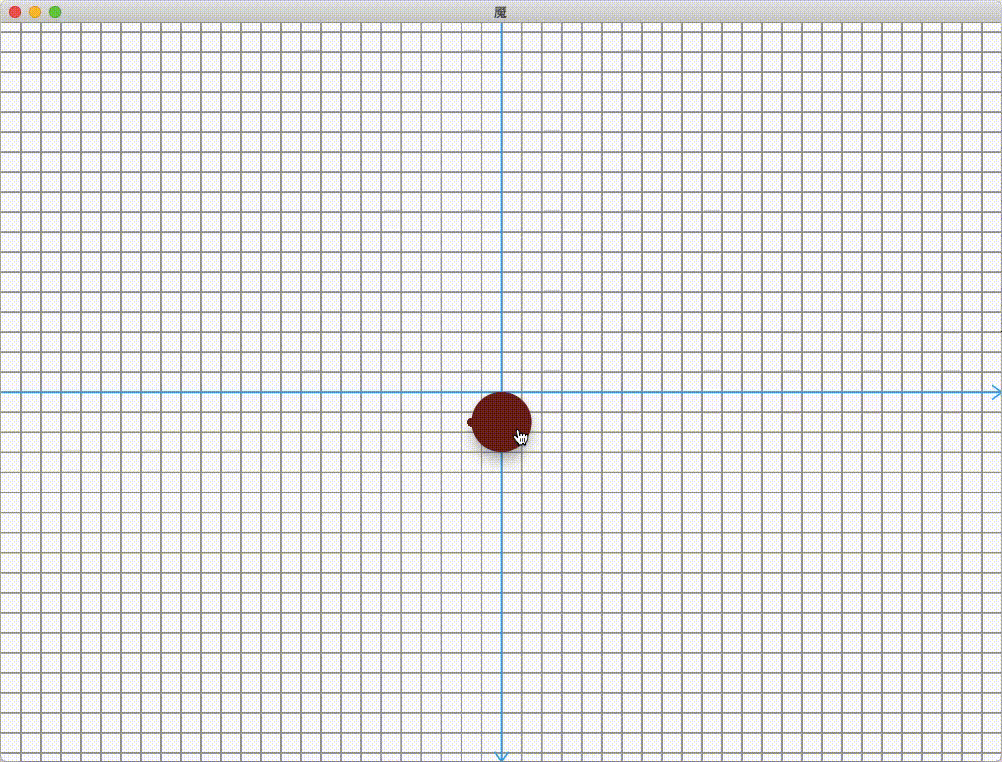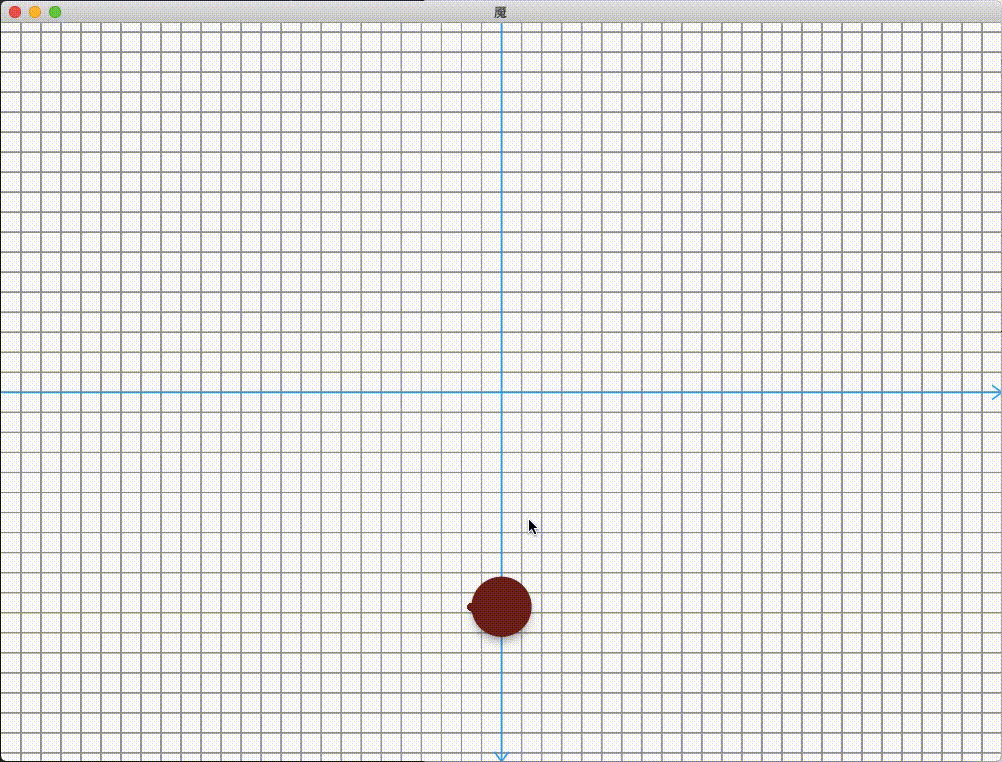4、实现第三、四部分动画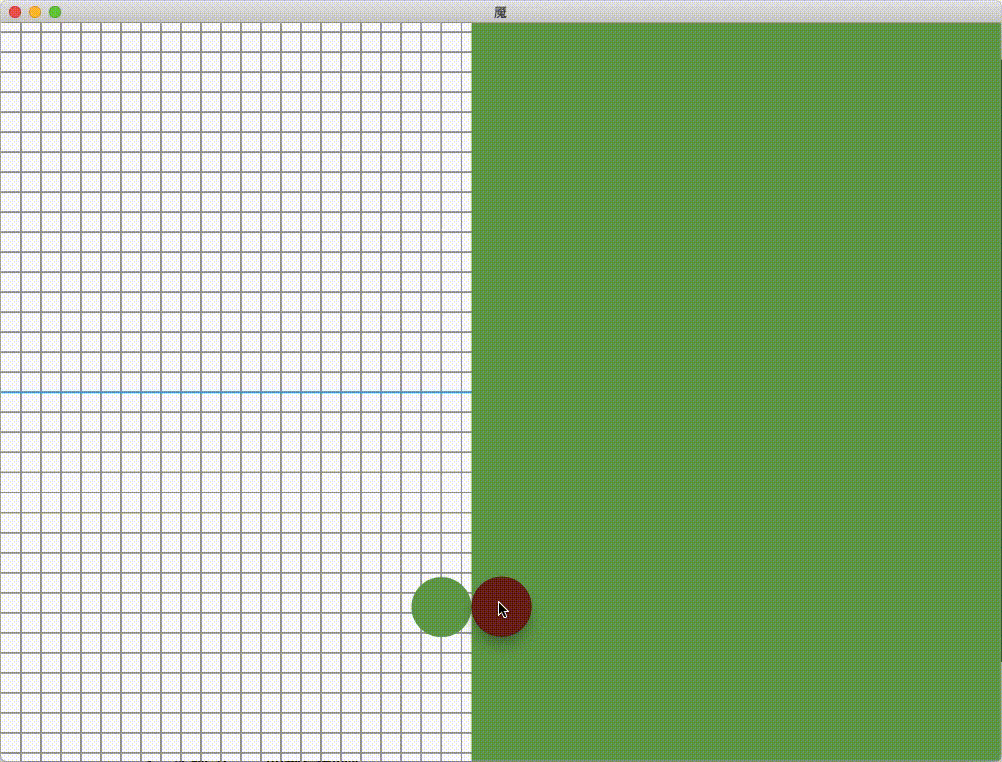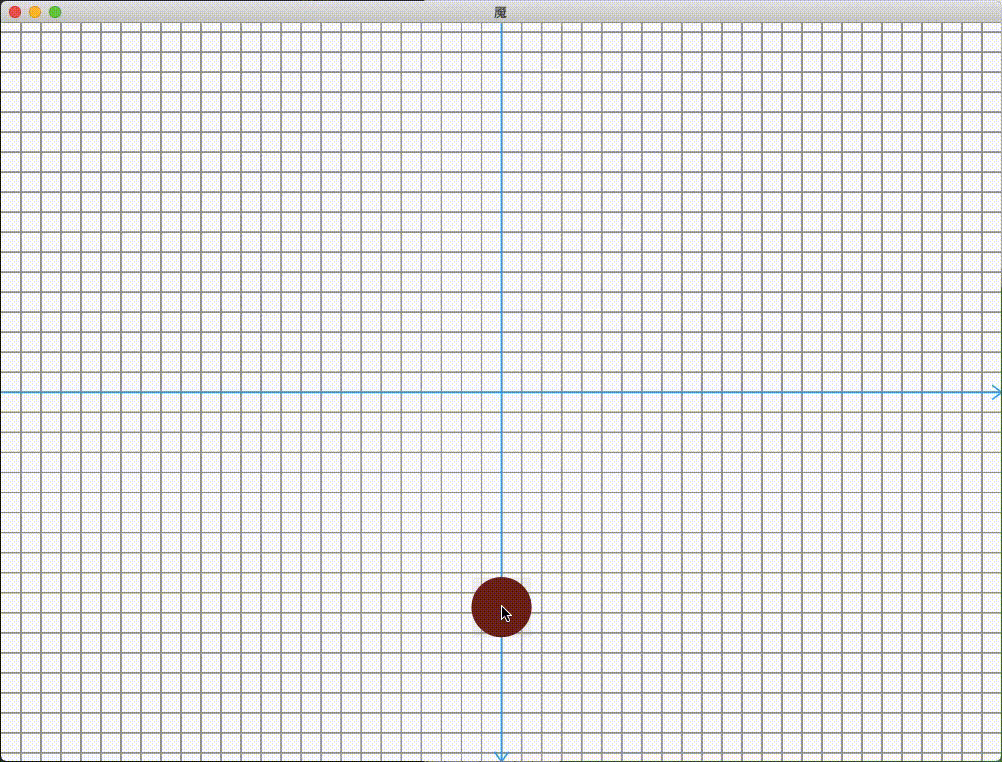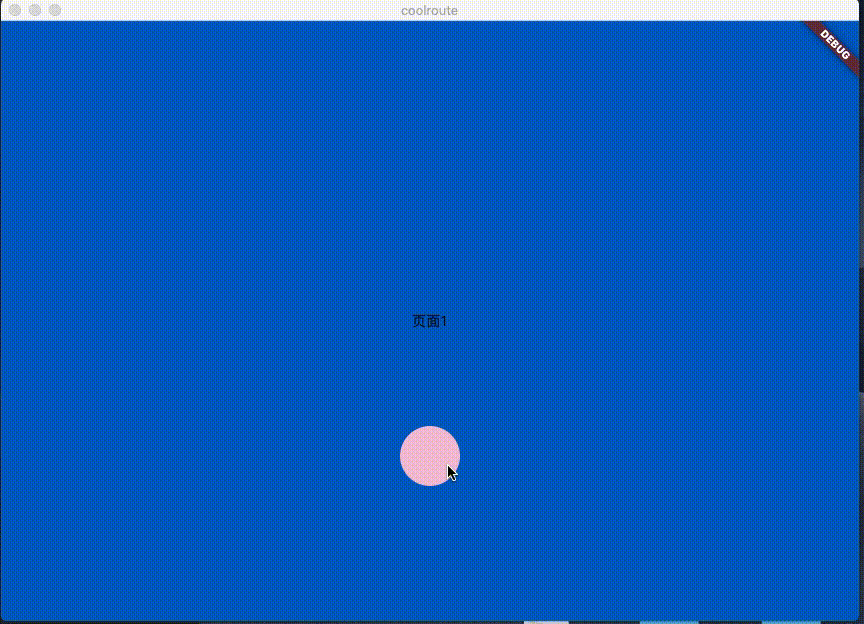ffmpeg 在转换的时候 gif 变慢了不知道为啥。另外路由前页面的位移动画，与路由后的页面位移动画需要另外加了 😑 。

四、组件封装

CoolButton(
curPageAccentColor: Color(0xff013bca),
buttonColor: Color(0xfffcb7d6),
nextButtonColor: Colors.white,
pushPage: PushPage(),
),

demo 地址：

MYS_Flutter

五、结语

• 代码写得比较赶，很多地方不规范，我在将这个路由引入自己项目后会改进。
• 上一篇“ 炫酷的波纹路由动画 ”是今年 2 月发布的，与现在对比了写文章的格式与排版，也算看到了自己这五个月在这方面的进步。
• “ MYS_Flutter ”初次创建是在两年前了，里面的其他 demo 的最后一次更新也是两年前😶 。
• 自己手上没有各种各样的项目，所以在学 Flutter 的时候不需要整天组各种各样的轮子，对我而言，我永远喜欢尝试新的东西，这也是我尝试了 Flutter 的原因。

happy coding !